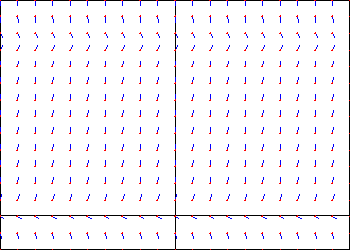# Solution of a Differential Equation?

## Homework Statement

The slope field for the equation $\frac{dP}{dt} = 0.0333P(30−P)$, for $P\geq0$, is shown below.What is the equation of the solution to this differential equation that passes through (0,0)?

## The Attempt at a Solution

I'm completely clueless as to how this is to be done. I tried using separable differential equations to solve this, but it didn't give me the right answer as I submitted it, the the website (this question comes from my online homework) said it was wrong. I would like someone to give me a hint as to how to approach this problem, and then I'll proceed with my attempt, thanks.

Last edited:

LCKurtz
Homework Helper
Gold Member

## Homework Statement

The slope field for the equation $\frac{dP}{dt} = 0.0333P(30−P)$, for $P\geq0$, is shown below.
View attachment 41087
What is the equation of the solution to this differential equation that passes through (0,0)?

## The Attempt at a Solution

I'm completely clueless as to how this is to be done. I tried using separable differential equations to solve this, but it didn't give me the right answer as I submitted it, the the website (this question comes from my online homework) said it was wrong. I would like someone to give me a hint as to how to approach this problem, and then I'll proceed with my attempt, thanks.

Separation of variables should work. It's hard to guess what went wrong unless you show us your work.

[Edit - added] Note the direction field itself gives a large hint.

Last edited:
Well I used partial fractions to solve this differential equation and I got this:
$-ln(\frac{|P-30|}{P}) = t + C$

If I try to solve for C using the initial condition (0,0), then I get that $-ln(\frac{30}{0}) = C$, which is undefined. If C is undefined, then what do you do?

LCKurtz
Homework Helper
Gold Member
Well I used partial fractions to solve this differential equation and I got this:
$-ln(\frac{|P-30|}{P}) = t + C$

If I try to solve for C using the initial condition (0,0), then I get that $-ln(\frac{30}{0}) = C$, which is undefined. If C is undefined, then what do you do?

Yes, you do run into a problem with separation of variables, which is why I added the hint. The problem is that as soon as you divide by P(30-P) you are disallowing the solutions P identically 0 or P identically 30. Yet if you check the original equation you will find that they work. And one of them satisfies your initial conditions. Look at your direction field and see if those don't look like solutions.

Last edited:
Wait how am I suppose to generate an equation from looking at the differential field?

HallsofIvy
Homework Helper
Unfortunately, that slope field is misleading. At (0,0), it appears that the slope is slightly negative but it clearly is not. If P= 0, dP/dt= 0.0333(0)(30- 0)= 0.
Now, what is the simplest function that is 0 at t= 0 and has derivative 0 there? What is its derivative for all t?

Okay well I tried P = 0 and it worked; I knew this could be an answer but that it couldn't have been this simple.HallsofIvy